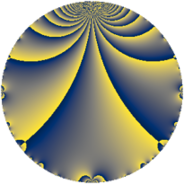# Properties

 Label 15.2.a.aLevel $15$ Weight $2$ Character orbit 15.a Self dual yes Analytic conductor $0.120$ Analytic rank $0$ Dimension $1$ CM no Inner twists $1$

# Learn more

## Newspace parameters

 Level: $$N$$ $$=$$ $$15 = 3 \cdot 5$$ Weight: $$k$$ $$=$$ $$2$$ Character orbit: $$[\chi]$$ $$=$$ 15.a (trivial)

## Newform invariants

 Self dual: yes Analytic conductor: $$0.119775603032$$ Analytic rank: $$0$$ Dimension: $$1$$ Coefficient field: $$\mathbb{Q}$$ Coefficient ring: $$\mathbb{Z}$$ Coefficient ring index: $$1$$ Twist minimal: yes Fricke sign: $$-1$$ Sato-Tate group: $\mathrm{SU}(2)$

## $q$-expansion

 $$f(q)$$ $$=$$ $$q - q^{2} - q^{3} - q^{4} + q^{5} + q^{6} + 3q^{8} + q^{9} + O(q^{10})$$ $$q - q^{2} - q^{3} - q^{4} + q^{5} + q^{6} + 3q^{8} + q^{9} - q^{10} - 4q^{11} + q^{12} - 2q^{13} - q^{15} - q^{16} + 2q^{17} - q^{18} + 4q^{19} - q^{20} + 4q^{22} - 3q^{24} + q^{25} + 2q^{26} - q^{27} - 2q^{29} + q^{30} - 5q^{32} + 4q^{33} - 2q^{34} - q^{36} - 10q^{37} - 4q^{38} + 2q^{39} + 3q^{40} + 10q^{41} + 4q^{43} + 4q^{44} + q^{45} + 8q^{47} + q^{48} - 7q^{49} - q^{50} - 2q^{51} + 2q^{52} - 10q^{53} + q^{54} - 4q^{55} - 4q^{57} + 2q^{58} - 4q^{59} + q^{60} - 2q^{61} + 7q^{64} - 2q^{65} - 4q^{66} + 12q^{67} - 2q^{68} - 8q^{71} + 3q^{72} + 10q^{73} + 10q^{74} - q^{75} - 4q^{76} - 2q^{78} - q^{80} + q^{81} - 10q^{82} + 12q^{83} + 2q^{85} - 4q^{86} + 2q^{87} - 12q^{88} - 6q^{89} - q^{90} - 8q^{94} + 4q^{95} + 5q^{96} + 2q^{97} + 7q^{98} - 4q^{99} + O(q^{100})$$

## Expression as an eta quotient

$$f(z) = \eta(z)\eta(3z)\eta(5z)\eta(15z)=q\prod_{n=1}^\infty(1 - q^{n})^{}(1 - q^{3n})^{}(1 - q^{5n})^{}(1 - q^{15n})^{}$$

## Embeddings

For each embedding $$\iota_m$$ of the coefficient field, the values $$\iota_m(a_n)$$ are shown below.

For more information on an embedded modular form you can click on its label.

Label $$\iota_m(\nu)$$ $$a_{2}$$ $$a_{3}$$ $$a_{4}$$ $$a_{5}$$ $$a_{6}$$ $$a_{7}$$ $$a_{8}$$ $$a_{9}$$ $$a_{10}$$
1.1
 0
−1.00000 −1.00000 −1.00000 1.00000 1.00000 0 3.00000 1.00000 −1.00000
 $$n$$: e.g. 2-40 or 990-1000 Significant digits: Format: Complex embeddings Normalized embeddings Satake parameters Satake angles

## Atkin-Lehner signs

$$p$$ Sign
$$3$$ $$1$$
$$5$$ $$-1$$

## Inner twists

This newform does not admit any (nontrivial) inner twists.

## Twists

By twisting character orbit
Char Parity Ord Mult Type Twist Min Dim
1.a even 1 1 trivial 15.2.a.a 1
3.b odd 2 1 45.2.a.a 1
4.b odd 2 1 240.2.a.d 1
5.b even 2 1 75.2.a.b 1
5.c odd 4 2 75.2.b.b 2
7.b odd 2 1 735.2.a.c 1
7.c even 3 2 735.2.i.e 2
7.d odd 6 2 735.2.i.d 2
8.b even 2 1 960.2.a.l 1
8.d odd 2 1 960.2.a.a 1
9.c even 3 2 405.2.e.f 2
9.d odd 6 2 405.2.e.c 2
11.b odd 2 1 1815.2.a.d 1
12.b even 2 1 720.2.a.c 1
13.b even 2 1 2535.2.a.j 1
15.d odd 2 1 225.2.a.b 1
15.e even 4 2 225.2.b.b 2
16.e even 4 2 3840.2.k.m 2
16.f odd 4 2 3840.2.k.r 2
17.b even 2 1 4335.2.a.c 1
19.b odd 2 1 5415.2.a.j 1
20.d odd 2 1 1200.2.a.e 1
20.e even 4 2 1200.2.f.h 2
21.c even 2 1 2205.2.a.i 1
23.b odd 2 1 7935.2.a.d 1
24.f even 2 1 2880.2.a.bc 1
24.h odd 2 1 2880.2.a.y 1
33.d even 2 1 5445.2.a.c 1
35.c odd 2 1 3675.2.a.j 1
39.d odd 2 1 7605.2.a.g 1
40.e odd 2 1 4800.2.a.bz 1
40.f even 2 1 4800.2.a.t 1
40.i odd 4 2 4800.2.f.bf 2
40.k even 4 2 4800.2.f.c 2
55.d odd 2 1 9075.2.a.g 1
60.h even 2 1 3600.2.a.u 1
60.l odd 4 2 3600.2.f.e 2

By twisted newform orbit
Twist Min Dim Char Parity Ord Mult Type
15.2.a.a 1 1.a even 1 1 trivial
45.2.a.a 1 3.b odd 2 1
75.2.a.b 1 5.b even 2 1
75.2.b.b 2 5.c odd 4 2
225.2.a.b 1 15.d odd 2 1
225.2.b.b 2 15.e even 4 2
240.2.a.d 1 4.b odd 2 1
405.2.e.c 2 9.d odd 6 2
405.2.e.f 2 9.c even 3 2
720.2.a.c 1 12.b even 2 1
735.2.a.c 1 7.b odd 2 1
735.2.i.d 2 7.d odd 6 2
735.2.i.e 2 7.c even 3 2
960.2.a.a 1 8.d odd 2 1
960.2.a.l 1 8.b even 2 1
1200.2.a.e 1 20.d odd 2 1
1200.2.f.h 2 20.e even 4 2
1815.2.a.d 1 11.b odd 2 1
2205.2.a.i 1 21.c even 2 1
2535.2.a.j 1 13.b even 2 1
2880.2.a.y 1 24.h odd 2 1
2880.2.a.bc 1 24.f even 2 1
3600.2.a.u 1 60.h even 2 1
3600.2.f.e 2 60.l odd 4 2
3675.2.a.j 1 35.c odd 2 1
3840.2.k.m 2 16.e even 4 2
3840.2.k.r 2 16.f odd 4 2
4335.2.a.c 1 17.b even 2 1
4800.2.a.t 1 40.f even 2 1
4800.2.a.bz 1 40.e odd 2 1
4800.2.f.c 2 40.k even 4 2
4800.2.f.bf 2 40.i odd 4 2
5415.2.a.j 1 19.b odd 2 1
5445.2.a.c 1 33.d even 2 1
7605.2.a.g 1 39.d odd 2 1
7935.2.a.d 1 23.b odd 2 1
9075.2.a.g 1 55.d odd 2 1

## Hecke kernels

This newform subspace is the entire newspace $$S_{2}^{\mathrm{new}}(\Gamma_0(15))$$.

## Hecke characteristic polynomials

$p$ $F_p(T)$
$2$ $$1 + T$$
$3$ $$1 + T$$
$5$ $$-1 + T$$
$7$ $$T$$
$11$ $$4 + T$$
$13$ $$2 + T$$
$17$ $$-2 + T$$
$19$ $$-4 + T$$
$23$ $$T$$
$29$ $$2 + T$$
$31$ $$T$$
$37$ $$10 + T$$
$41$ $$-10 + T$$
$43$ $$-4 + T$$
$47$ $$-8 + T$$
$53$ $$10 + T$$
$59$ $$4 + T$$
$61$ $$2 + T$$
$67$ $$-12 + T$$
$71$ $$8 + T$$
$73$ $$-10 + T$$
$79$ $$T$$
$83$ $$-12 + T$$
$89$ $$6 + T$$
$97$ $$-2 + T$$
show more
show less